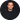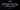Published on

# Writing the Same Program 10 Times. In 10 Different Languages.

Author
•Name
Robert Kirby
Title
Lead Full Stack Developer at Y-SquaredBlog Post 6

## Writing the Same Program 10 Times. In 10 Different Languages.

1. Introduction
2. C
3. JavaScript
4. Python
5. C++
6. Java
7. TypeScript
8. PHP
9. Swift
10. R
11. Go (Also Known as Golang)

## 1. Introduction

I wrote a simple program in JavaScript, then replicated it in 9 other programming languages so we can see a side by side comparison. This is not intended to be a language tutorial but rather just an interesting exercise to see how the same end goal is obtained across the different languages, this will allow us to see the differences and similarities clearly.

The program I’ve written for the examples in this post is a simple “Compound Interest Calculator”, It takes three inputs: 1. The initial amount 2. The yearly interest 3. The number of years.

## 2. C

C is a low level general-purpose programming language that was originally developed in the early 1970s. When we say low level it means it's closer to machine code and allows greater control over hardware.

The reason we are starting with C is that most of the big programming languages we work with today are built on top of C. For example, Python and Javascript are both built on top of C and are considered higher level languages. Higher level languages are easier to work with and understand and usually have a lot of built in functionality.

C Example:

``````#include <stdio.h>
#include <math.h>

double compoundInterest(double initial, double rate, double years) {
double final = initial * pow(1 + rate / 100, years);
return final;
}

int main() {
double final = compoundInterest(10000, 10, 10);
printf("%f\n", final);
return 0;
}

// Output: 25937.424601
// Run this code at https://www.tutorialspoint.com/online_c_compiler.php
``````

## 3. JavaScript

JavaScript is a programming language that is primarily used to add interactivity and dynamic features to websites. It was initially only used on the frontend development but in recent years can be used for almost anything for example with the addition of Node.js allowing backend development and TensorFlow.js for machine learning.

JavaScript Example:

``````function compoundInterest(initial, rate, years) {
let final = initial * Math.pow(1 + rate / 100, years);
return final;
}

let final = compoundInterest(10000, 10, 10);

console.log(final);

// Output: 25937.424601000024
// Run this code at https://jsfiddle.net/
``````

## 4. Python

Python is a high-level, interpreted programming language that is widely used for web development, data science and general-purpose programming.

Python Example:

``````#!/usr/bin/python3

def compoundInterest(initial, rate, years):
final = initial * (1 + rate / 100) ** years
return final

final = compoundInterest(10000, 10, 10)

print(final)

#  Output: 25937.424601000024
#  Run this code at https://www.sololearn.com/compiler-playground/cOAXyhEmN1f7
``````

## 5. C++

C++ is a high-level, statically typed, general-purpose programming language and is an extension of the C programming language.

C++ Example:

``````#include <iostream>
#include <cmath>

using namespace std;

double compoundInterest(double initial, double rate, double years) {
double final = initial * pow(1 + rate / 100, years);
return final;
}

int main() {
double final = compoundInterest(10000, 10, 10);
cout << final << endl;
return 0;
}

// Output: 25937.4
// Run this code at https://cpp.sh/
``````

## 6. Java

Java is a high-level object-oriented language programming language that is commonly used for building large-scale applications.

Java Example:

``````public class Main {
public static double compoundInterest(double initial, double rate, double years) {
double finalAnswer = initial * Math.pow(1 + rate / 100, years);
}

public static void main(String[] args) {
double finalAnswer = compoundInterest(10000, 10, 10);
}
}

// Output: 25937.424601000024
// Run this code at https://www.sololearn.com/compiler-playground/java
``````

## 7. TypeScript

TypeScript is a strongly typed superset of JavaScript with additional features. It can be used for anything JavaScript is used for and is growing in popularity as its strong typing and other features make it easier to develop and maintain large applications.

TypeScript Example:

``````const ci = function compoundInterest(
initial: number,
rate: number,
years: number
): number {
let result = initial * Math.pow(1 + rate / 100, years);
return result;
};
console.log(ci(10000, 10, 10));

// Output: 25937.424601000024
// Run this code at https://www.typescriptlang.org/play
``````

## 8. PHP

PHP is a server-side scripting language that is commonly used for web development. It is a popular choice for developing dynamic websites and web applications. PHP is often used in combination with other technologies to create powerful web applications.

PHP Example:

``````<?php

function compoundInterest(\$initial, \$rate, \$years) {
\$final = \$initial * pow(1 + \$rate / 100, \$years);
return \$final;
}

\$final = compoundInterest(10000, 10, 10);

echo \$final;

// Output: 25937.424601
// Run this code at https://onlinephp.io/
``````

## 9. Swift

SWIFT is a programming language developed by Apple based on the C and Objective-C and is used mainly to build apps for apple operating systems like IOS and MacOS.

Swift Example:

``````func compoundInterest(initial: Double, rate: Double, years: Double) -> Double {
let final = initial * pow(1 + rate / 100, years)
return final
}

let final = compoundInterest(initial: 10000, rate: 10, years: 10)

print(final)

// Output: 25937.424601000024
// Run this code at https://swiftfiddle.com/
``````

## 10. R

R is a programming language and software environment for statistical computing. It is widely used across data science.

``````compoundInterest <- function(initial, rate, years) {
final <- initial * (1 + rate / 100)^years
return(final)
}

final <- compoundInterest(10000, 10, 10)

print(final)

# Output: 25937.42
#  Run this code at https://rdrr.io/snippets/
``````

## 11 Go (Also Known as Golang)

Go is a statically typed general purpose programming language developed by Google.

Go Example:

``````import (
"fmt"
"math"
)

func compoundInterest(initial float64, rate float64, years float64) float64 {
final := initial * math.Pow(1 + rate/100, years)
return final
}

func main() {
final := compoundInterest(10000, 10, 10)
fmt.Println(final)
}

// Output: 25937.424601000017
// Run this code at https://go.dev/play/
``````

Please fill out the form on https://y-squared.com/contact or email us at team@y-squared.com.

The Author:This post was written by Robert from Y-Squared:

Blog Site:

https://y-squared.blog/